• 限制性立方样条回归
2022-04-08 22:13:14

# R语言使用cph函数和rcs函数构建限制性立方样条cox回归模型、使用anova函数进行方差分析通过p值确认指定连续变量和风险值HR之间是否存在非线性关系

目录数据挖掘 人工智能 机器学习 r语言
更多相关内容
• R语言生存分析之限制性立方样条分析：为什么需要样条分析、样条回归、和多项式回归有什么不同？是否存在非线性关系、限制性立方样条cox回归模型

# R语言生存分析之限制性立方样条分析：为什么需要样条分析、样条回归、和多项式回归有什么不同？是否存在非线性关系、限制性立方样条cox回归模型

目录

展开全文数据挖掘 人工智能 机器学习 r语言
• 限制性立方样条函数 (restricted cubic spline,RCS) 属于多项式中的一种，最大的特点在于它进行了样条插值并对趋势首尾两端进行了线性限制，即在第一个节点前的趋势和最后一个节点后的趋势强制限制为线性。...

### 限制性立方样条（Restricted Cubic Spline）

在上一篇文章等时替代模型的案例论文中，我们可以看到，COX比例风险回归模型中的连续变量可能会对生存时间产生非线性风险，如果不考虑这个风险，就会对结果造成偏差。非线性关系的拟合思路是在线性关系上加入高次项，如二次项和三次项，这样就构成了高次多项式模型。因此，作者对于非线性趋势的拟合采用了限制性立方样条(restricted cubic spline,RCS)的方法，其中也简单提到了，RCS是用来拟合自变量和因变量之间非线性关系的一个好方法。

限制性立方样条函数属于多项式中的一种，最大的特点在于它进行了样条插值并对趋势首尾两端进行了线性限制，即在第一个节点前的趋势和最后一个节点后的趋势强制限制为线性。在这篇文章中，笔者就将对限制性立方样条的原理和案例进行总结。

# 一、背景

## （一）什么是线性 (Linearity) ？

维基百科的定义：

Linearity is the property of a mathematical relationship (function) that can be graphically represented as a straight line. 1
线性是指可以用图形表示为直线的数学关系或函数

Additivity: f(x + y) = f(x) + f(y).
Homogeneity of degree 1: f(αx) = α f(x) for all α.

对于多维函数的推广，线性是指函数兼容加法和定标的性质，也称为叠加原理

## （二）为什么要做线性假设？

线性是建立线性回归模型时的常见假设。

因为现实世界里面许多量之间具有线性或者近似线性的依赖关系，即使不是线性关系，我们对变量进行适当的变换，得到的新的变量可能也会具有近似的线性关系。一旦问题落脚到线性问题，那在数学里面积累的大量的、丰富的处理线性关系的理论和方法就能用上了。

而且线性模型具有一个最大的优势，就是易于解释。在线性关系中，连续变量在整个变量范围内具有相同的影响。

例如，在传统的等时替代模型中，我们用系数b代表替代效应。系数b1就表示在保持总体活动时间不变的情况下，用其他活动去替换x1的时候，对健康结果变量y的估计效果。

这很容易理解，线性使得估计易于解释，也让传统的模型更具有优势：模型中每增加一个单位就会给出相应系数在结果中的变化。

但是，要知道，自然界中的规律很难会遵循一条简单的直线。对于今天的这些复杂的大型数据集，我们的模型应该允许，也必须允许非线性效应的存在。

## （三）如何面对非线性的难题？

有许多非线性替代方案可以帮助我们去面对这些非线性的难题，从而更好地找到变量之间的联系。
这些替代方案大多数都依赖于将单个连续变量转换为多个。其中最简单的方法就是把变量转换为多项式。

例如，下面这个模型：我们可以对年龄这个变量进行扩展，让它还包括年龄的平方：变成多项式后，所得的就是曲线了。这样就产生了一个问题，当我们添加平方项后，前面的系数就变得难以解释，并且如果我们再添加三次项，即Age³，那前面的系数就几乎不可能解释了。
正是由于这种解释困难，我们要么使用 rms::contrast 函数，要么使用stats::predict来说明变量的行为方式。

## （四）多项式回归

本篇文章的案例数据均来自下面随机生成的数据：

options(max.print=25)
set.seed(76)

f <- function(x){
f_x <- 0.2*x^11*(10*(1-x))^6+10*(10*x)^3*(1-x)^10
return(f_x)
}

values <- data_frame(
x = seq(from=0, to=1, length=500),
f_x = f(x),
eps = rnorm(n=500, mean=0, sd=2),
y = f_x + eps
)

values %>%
ggplot(aes(x=x)) +
stat_function(fun = f) +
geom_point(aes(y=y))


对于函数f，在范围（0,1）上按照期望为0，方差为2的正态分布，随机产生500个噪点，结果如下图：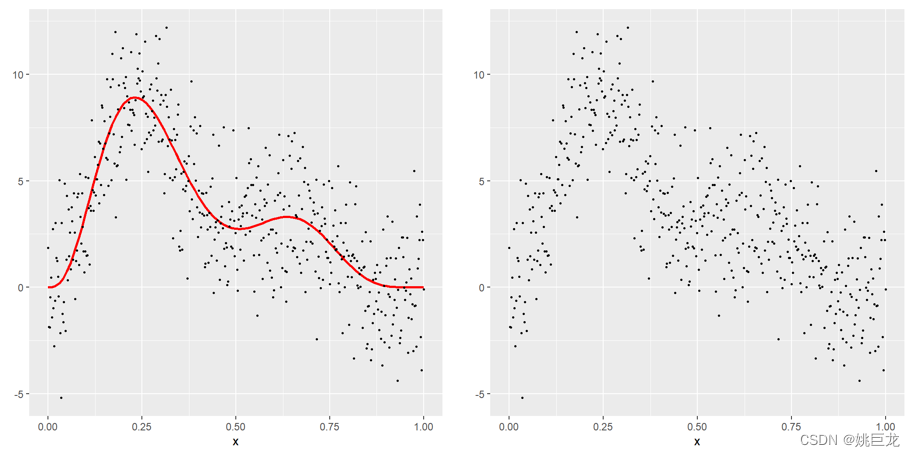对噪点分别进行一次、二次、三次、四次多项式回归：

ggplot(values,aes(x=x,y=y))+geom_point()

lmp1=lm(y~poly(x,1),data = values)
poly1=predict(lmp1,values)
values$poly1=poly1 lmp2=lm(y~poly(x,2),data = values) poly2=predict(lmp2,values) values$poly2=poly2

lmp3=lm(y~poly(x,3),data = values)
poly3=predict(lmp3,values)
values$poly3=poly3 lmp4=lm(y~poly(x,4),data = values) poly4=predict(lmp4,values) values$poly4=poly4

valueslong=gather(values,key="model",value = "value",
c("poly1","poly2","poly3","poly4"))
ggplot(valueslong)+theme_bw()+geom_point(aes(x,y))+
geom_line(aes(x=x,y=value,linetype=model,colour=model),size=1.0)+
theme_update(axis.title.y=element_blank())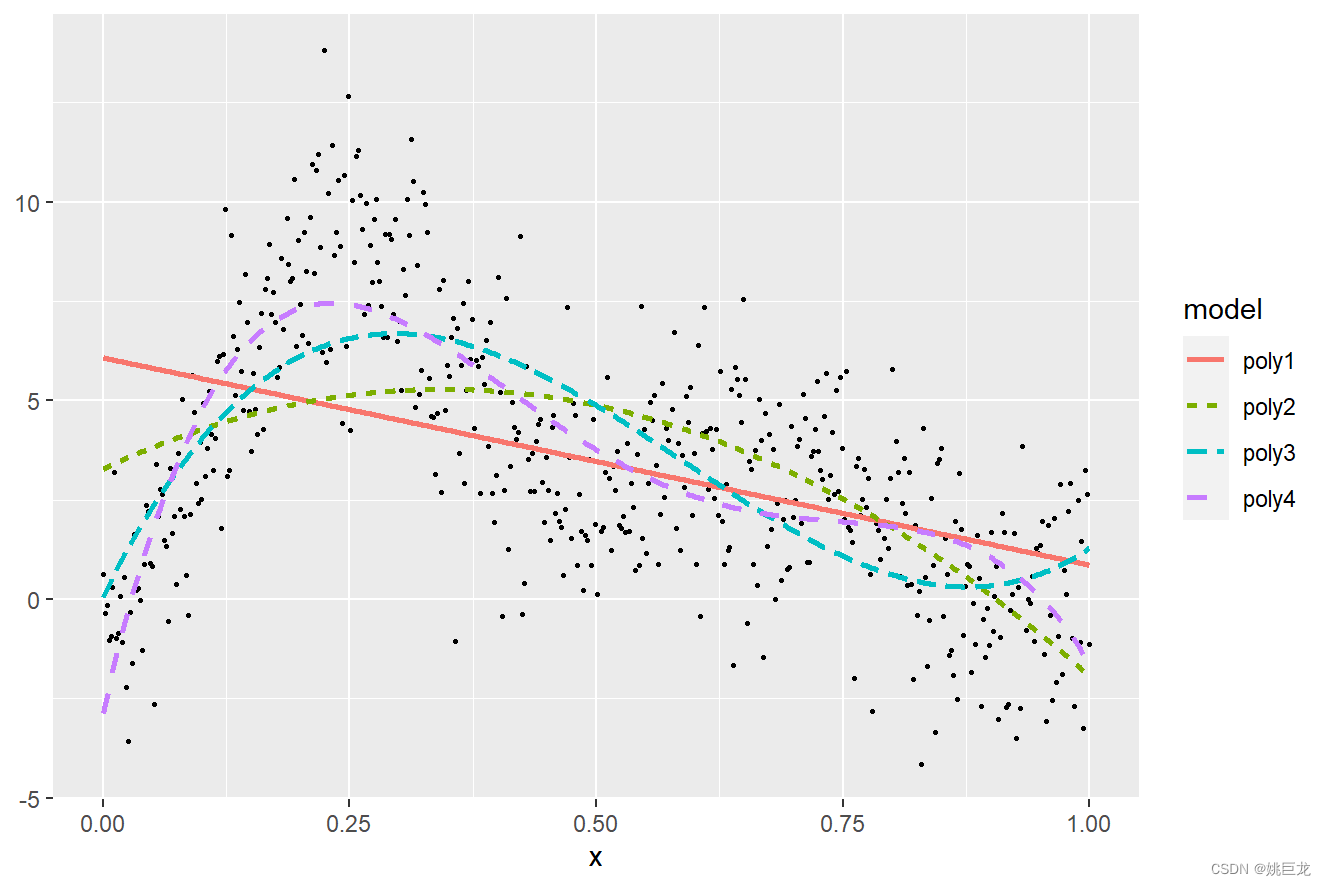多项式的使用是一种粗略的估算方法，具有可以产生平滑拟合的优点，但是它所拟合的函数也十分复杂，最大的缺点就是不灵活。

例如，二次曲线的形状必须是“U”形（或倒“U”形）——上升的必须以相同的速度下降；多项式通常会导致曲线在末端不能很好地拟合数据。

因此，对于多项式，我们也需要找到更好的方法去替代它，本篇文章所要介绍的方法——样条曲线 (Spline) ，就是一类灵活的替代函数。

# 二、样条函数

## （一）样条 (Spline) 的定义

### 1. 原始定义

样条（spline）原本是指工匠的工具，它是一种灵活的细木条或金属条，用来绘制平滑曲线。一般会用几个重物施加在工具的不同位置，让条带根据重物的数量和位置发生弯曲。### 2. 数学定义

类似的作用，样条曲线用于统计，是为了方便在数学上重现灵活的形状。样条曲线本质是一个分段多项式函数，此函数受限于某些控制点，称为 “节点”，节点放置在数据范围内的多个位置，多项式的类型以及节点的数量和位置决定了样条曲线的类型。

样条函数是一组平滑连接的分段多项式。 “平滑连接”意味着对于 n 次多项式，样条函数及其前 n-1 阶导数在节点处都是连续的。

## （二）节点的选择

### 1. 节点 (knots)

如果使用 k 个结，拟合 n 次多项式需要 k + n + 1 个回归参数（包括截距）。

在实践中，通常使用三次样条（即 3 次多项式），除截距外还需要 k + 3 个系数，而拟合线性模型只需 1 个系数。 这是允许拐点的多项式的最小度数，为拟合数据提供了足够的灵活性，同时不像高阶样条那样需要那么多的自由度。 由于一阶和二阶导数（斜率和斜率的变化率）在节点处是连续的，三次样条曲线在外观上是平滑的2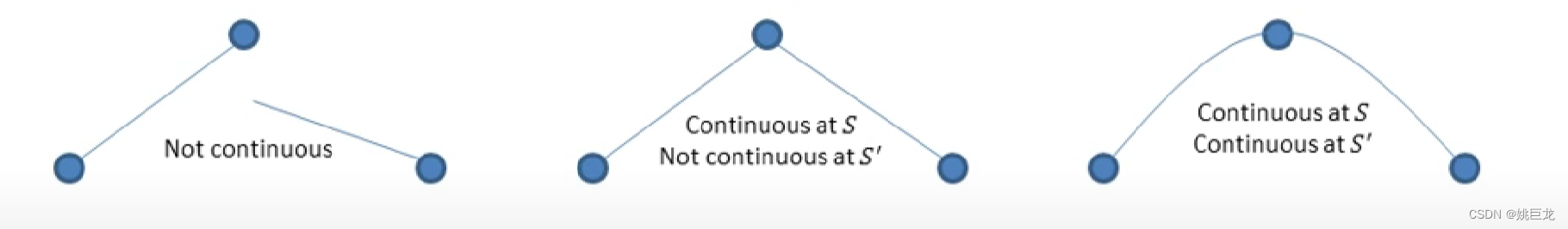### 2. R软件拟合示例

R示例：

library(tidyverse)
library(broom)

df_values <- c(2, 5, 10, 15, 25, 50)
overall <- NULL

for(df in df_values){
overall <- smooth.spline(values$x, values$y, df=df) %>%
augment() %>%
mutate(df=df) %>%
bind_rows(overall)
}
multiple_df <- overall %>%
ggplot(aes(x=x)) +
geom_point(aes(y=y)) +
geom_line(aes(y=.fitted)) +
facet_wrap(~df, nrow=2) +
labs(title="Splines fit with different degrees of freedom")

multiple_df +
stat_function(fun = f)


如下图所示，是节点分别为2、5、10、15、25、50时，利用R软件，对黑色噪点进行拟合得到的图形。（蓝色是拟合曲线，红色是真实曲线）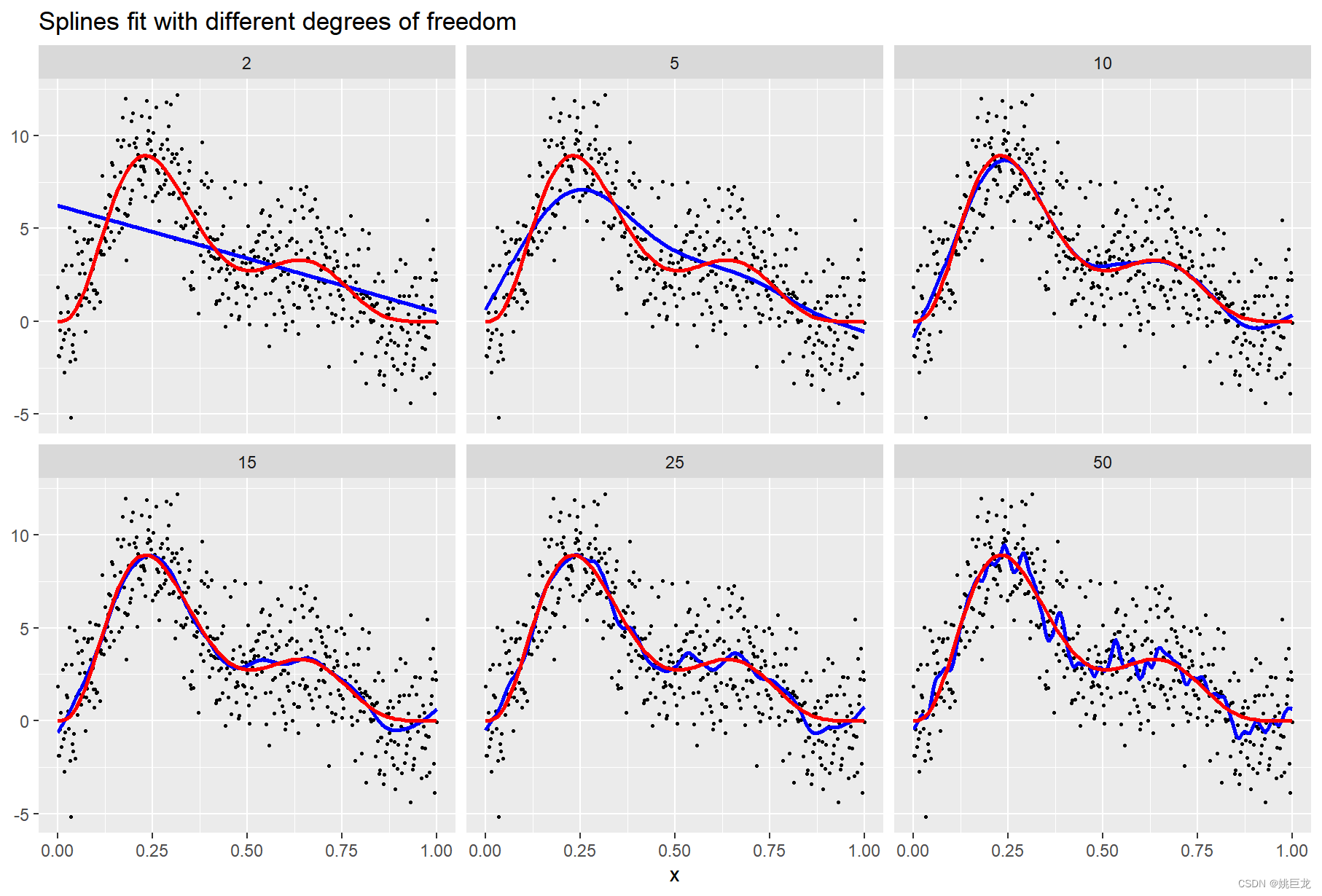## （三）样条的分类

### 1. B-splines（B样条/多次回归样条）

如果样条是基曲线的线性组合， 则称为B样条(B-spline)
基函数为：B-spline定义为：### 2. Natural splines（自然样条/限制性立方样条）‘Natural Cubic Spline’ — is a piece-wise cubic polynomial that is twice continuously differentiable. It is considerably ‘stiffer’ than a polynomial in the sense that it has less tendency to oscillate between data points.# 三、限制性立方样条

## （一）定义

回归样条(regression spline)本质上是一个分段多项式,但它一般要求每个分段点上连续并且二阶可导。

即:设自变量数据的范围在区间[a,b]，并根据需要分成k个段：a = t0 < t1 < … < tk-1 < tk = b，在每个区间 [ti-1,ti) 分别用一个多项 Si(x) 式表示，则回归样条 f(x) = Si(x) 当 x∈[ ti-1,ti )，并且 f ″(x) 在 [a,b] 存在连续3

限制性立方样条 (restricted cubic spline,RCS) 是在回归样条的基础上附加要求：样条函数在自变量数据范围两端的两个区间 [t0,t1) 和 (tk-1,tk] 内是线性函数，满足上述性质的样条函数通常用 RCS(X) 表示，其表达式为：更简单地一种理解：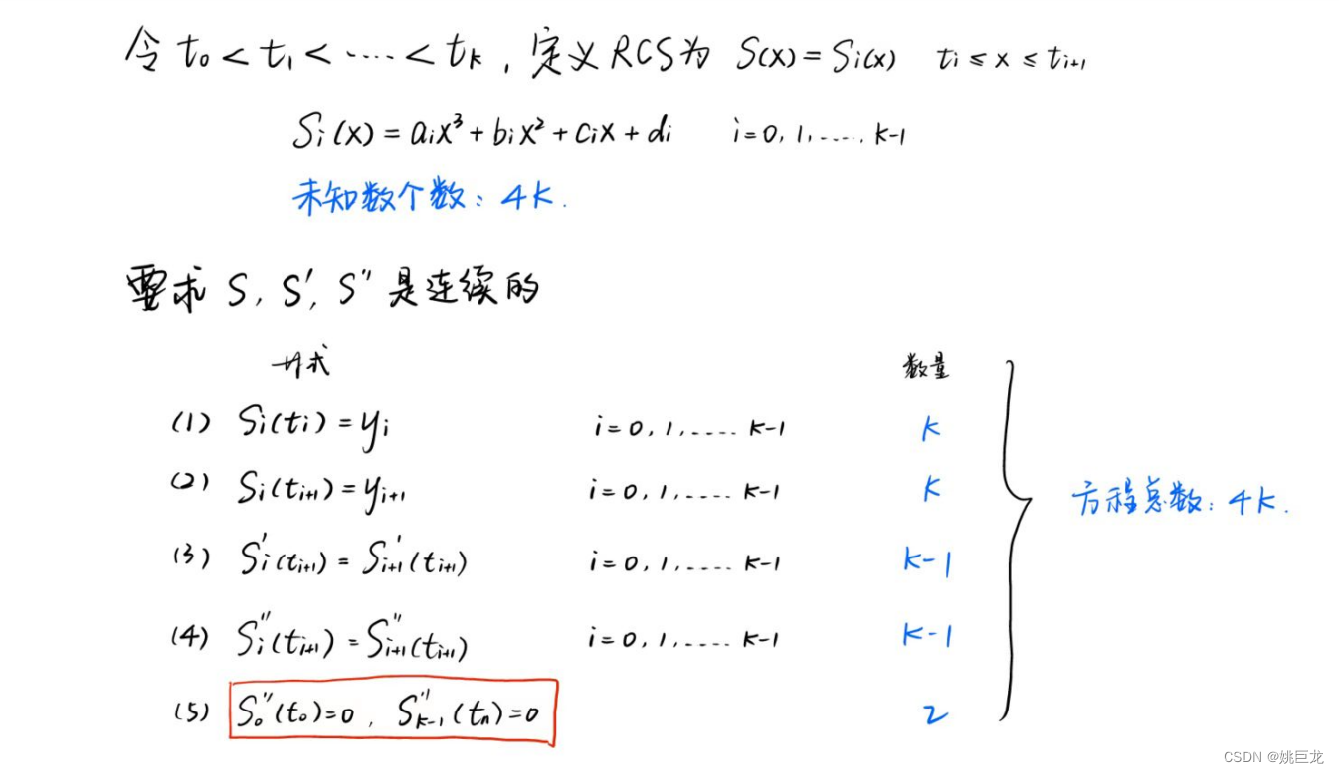## （二）RCS节点的数量和位置

结的数量比位置更重要。有研究表明，五个结足以很好地适应实践中可能出现的任何模式。

Harrell (2010) 指出“对于许多数据集，k = 4 提供了模型的充分拟合，并且是在灵活性和由过度拟合小样本引起的位置损失之间的良好折衷”4

• 如果样本量很小，则应使用三个节点，以便在节点之间有足够的观测值，以便能够拟合每个多项式。
• 如果样本量很大并且有理由相信所研究的关系变化很快，则可以使用超过 5 个节点。

对于一些研究，理论可能会暗示结的位置。更常见的是，节点的位置是根据连续变量的分位数预先指定的，这确保了每个区间中有足够的观测值来估计三次多项式。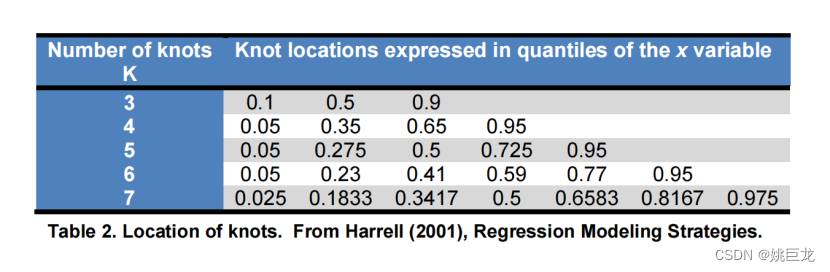## （三）R软件rms程序包操作示例

x = seq(from=0, to=1, length=500)
y = f(x)
model <- lm(y ~ x)

# restricted cubic spline
model.spline1 <- lm(y ~ rcs(x, 3))
model.spline2 <- lm(y ~ rcs(x, 5))
model.spline3 <- lm(y ~ rcs(x, 7))
model.spline4 <- lm(y ~ rcs(x, 9))
model.spline5 <- lm(y ~ rcs(x, 10))

# compare models (to determine number of knots)
AIC(model.spline1,model.spline2,model.spline3,model.spline4,model.spline5)



比较不同节点数对应的AIC值：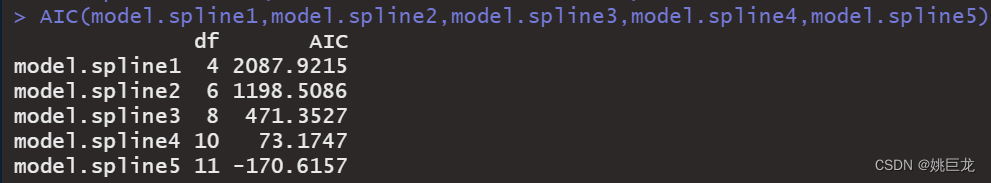ggplot(data=values, aes(x=x, y=y))  +
geom_point()+geom_smooth(aes(x=x, y=y),
method="lm", formula=y ~ rcs(x, 3),
se=FALSE, colour="blue") +
geom_point()+geom_smooth(aes(x=x, y=y),
method="lm", formula=y ~ rcs(x, 5),
se=FALSE, colour="red") +
geom_point()+geom_smooth(aes(x=x, y=y),
method="lm", formula=y ~ rcs(x, 9),
se=FALSE, colour="orange")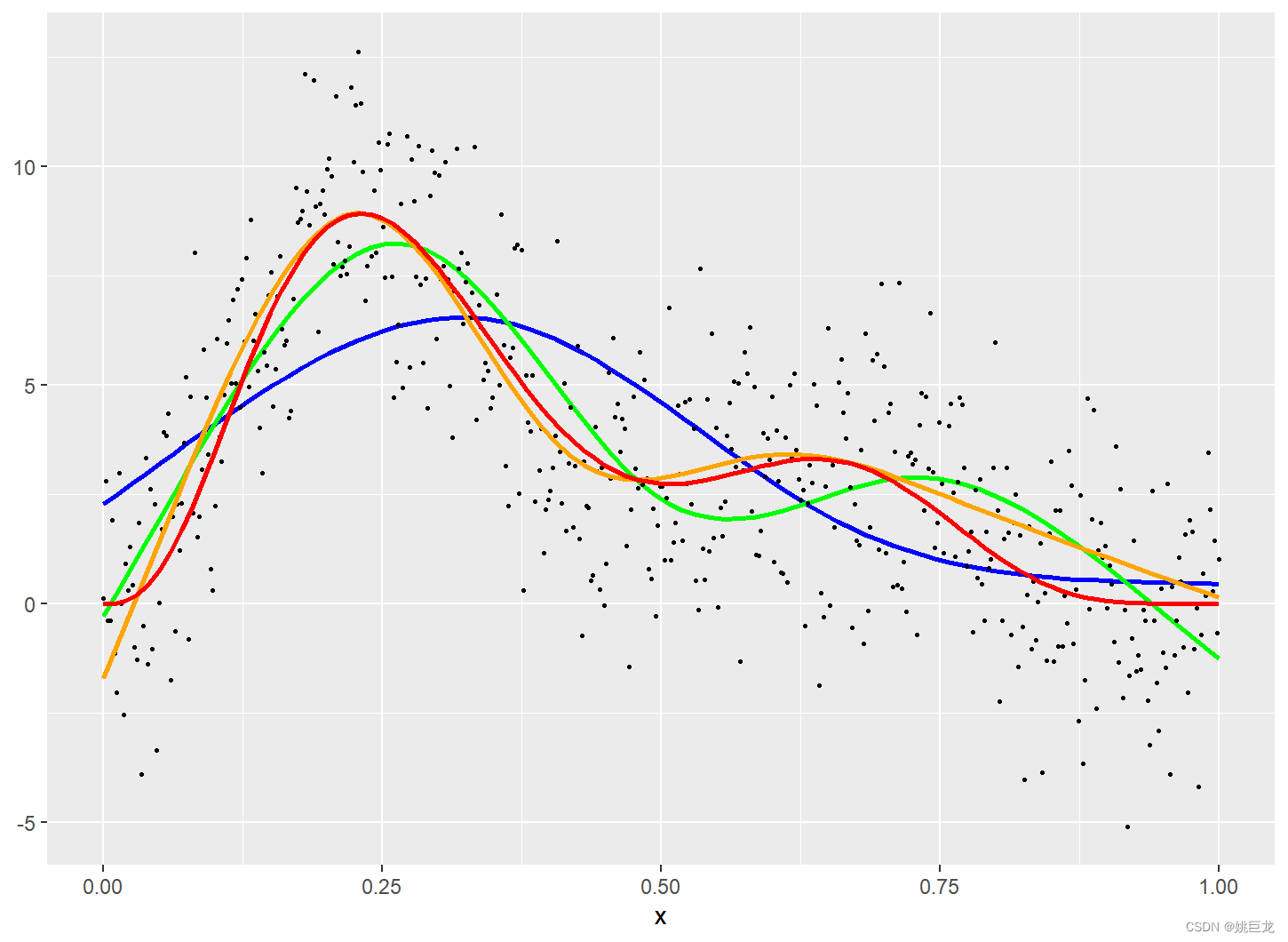# 四、相关文献阅读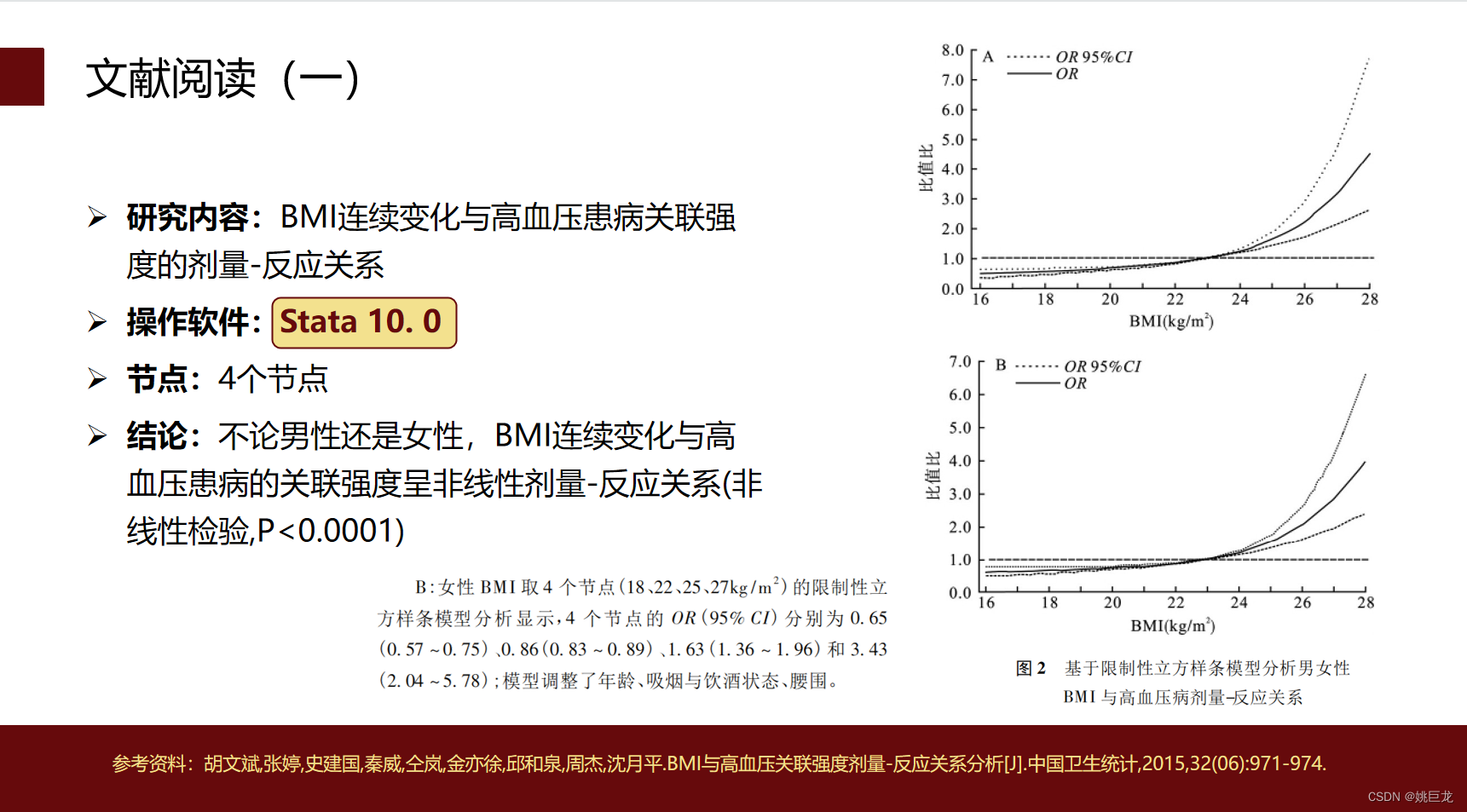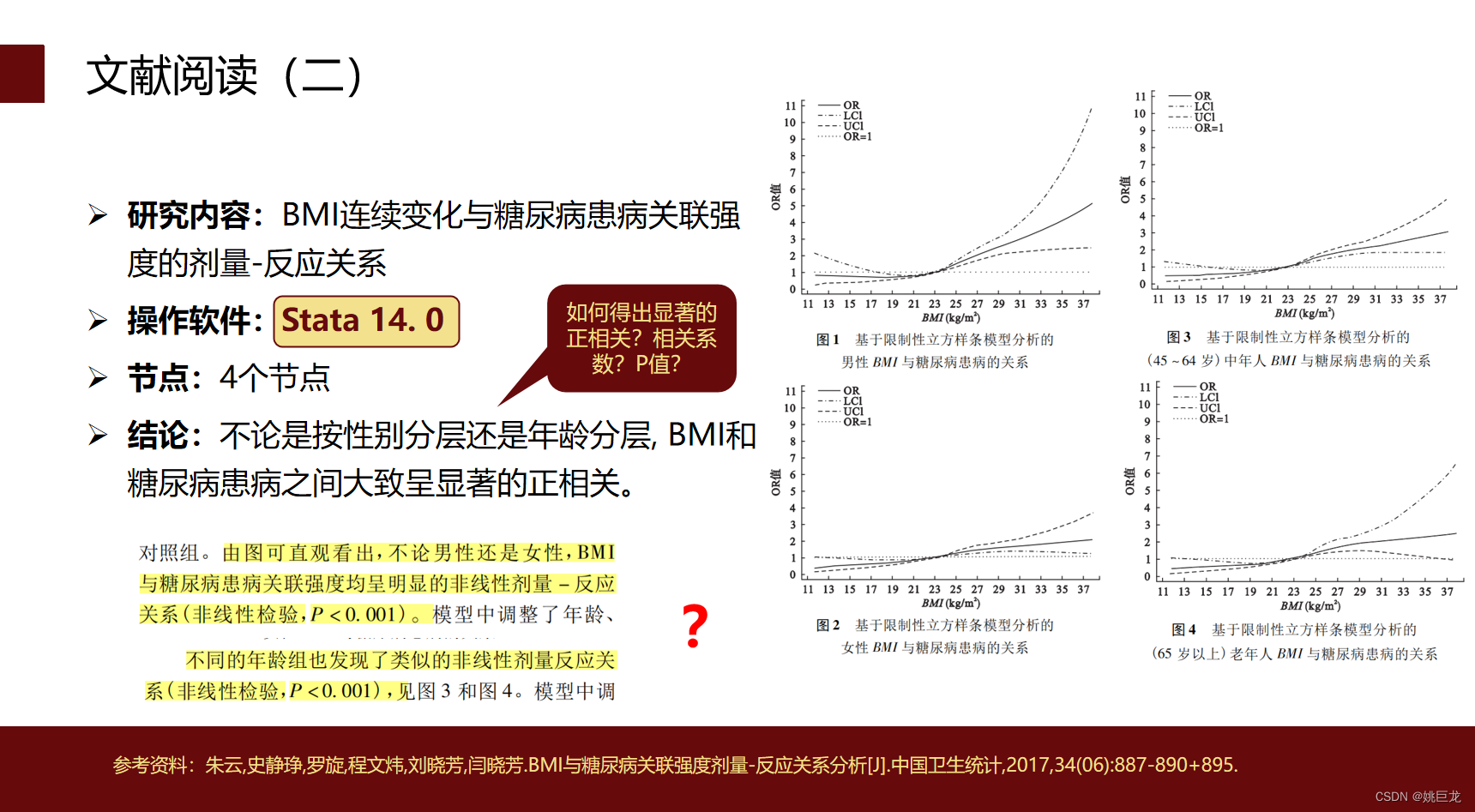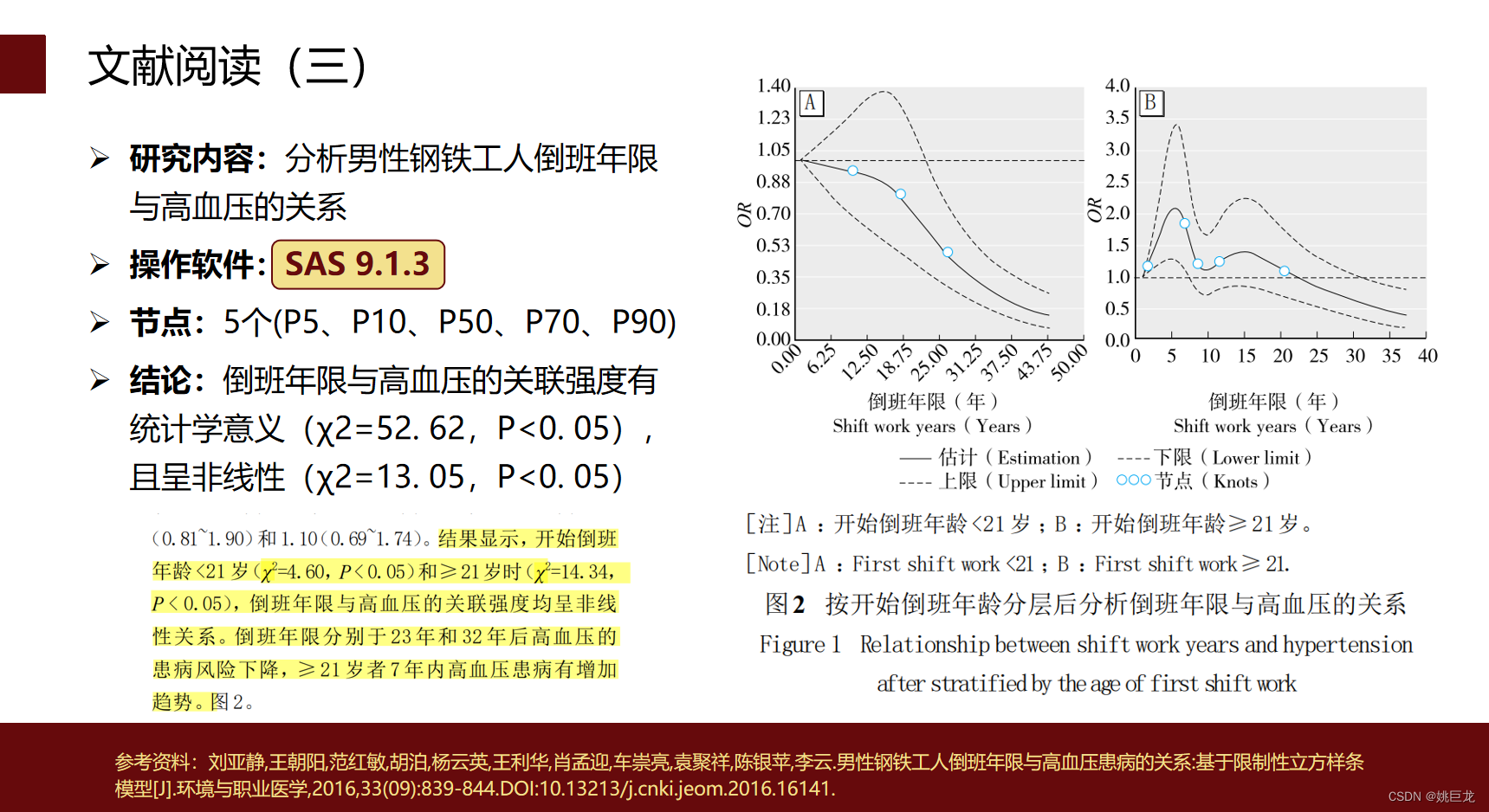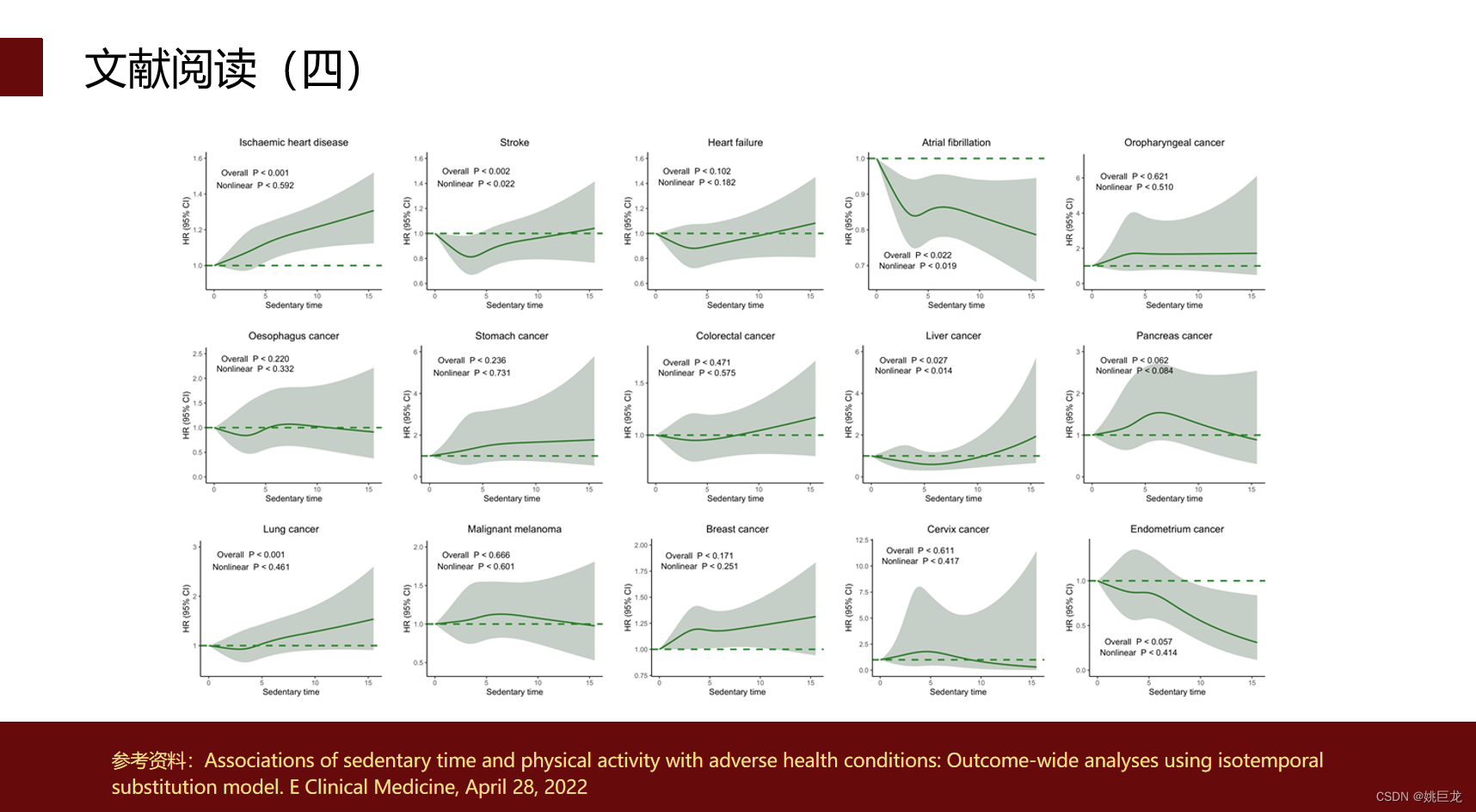# 参考文献

1. 维基百科：https://en.wikipedia.org/wiki/Linearity ↩︎

2. Croxford, Ruth. “Restricted Cubic Spline Regression : A Brief Introduction.” (2016). ↩︎

3. 罗剑锋,金欢,李宝月,赵耐青.限制性立方样条在非线性回归中的应用研究[J].中国卫生统计,2010,27(03):229-232. ↩︎

4. Harrell, F.E. (2010) Regression Modeling Strategies: with applications to linear models, logistic regression, and survival analysis. Springer-Verlag New York, Inc. New York, USA. ↩︎

展开全文多项式回归 限制性立方样条 R语言
• 我用stata跑出来的限制性立方样条图类似"✓"型 但是用testparm指令跑出来的非线性检验Pnon-linearity值却>0.05，即认为是线性 两个对应不起来是什么原因呢？ 感谢大家帮助逻辑回归
• 当自变量和因变量之间为非线性关系时，可以将连续型变量转化为分类变量，但是分类变量的类别数目以及节点位置的选择一般会带有主观并且分类变量会损失部分信息；也可以直接拟合自变量和因变量之间的非线性关系，...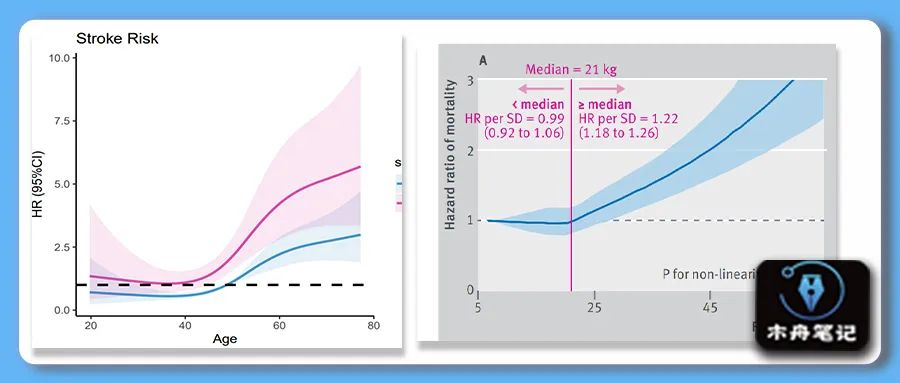在科学研究中，我们经常构建回归模型来分析自变量因变量之间的关系。大多数的回归模型有一个重要的假设就是自变量和因变量呈线性关联。当自变量和因变量之间为非线性关系时，可以将连续型变量转化为分类变量，但是分类变量的类别数目以及节点位置的选择一般会带有主观性并且分类变量会损失部分信息；也可以直接拟合自变量和因变量之间的非线性关系，但是直接构建多项式回归可能存在过度拟合、共线性等问题。因此，一个更好的解决方法是拟合自变量与因变量之间的非线性关系，「限制性立方样条」(Restricted cubic spline，RCS)就是分析非线性关系的最常见的方法之一。

样条（spline）原本是指是一种灵活的细木条或金属条，用来绘制平滑曲线。样条曲线本质是一个分段多项式函数，此函数受限于某些控制点，称为 “节点”，节点放置在数据范围内的多个位置，多项式的类型以及节点的数量和位置决定了样条曲线的类型。立方则指的是 函数为3次多项式。限制是在回归样条的基础上附加要求：样条函数在自变量数据范围两端的两个区间 [X1,X2)(Xn-1,Xn] 内是线性函数。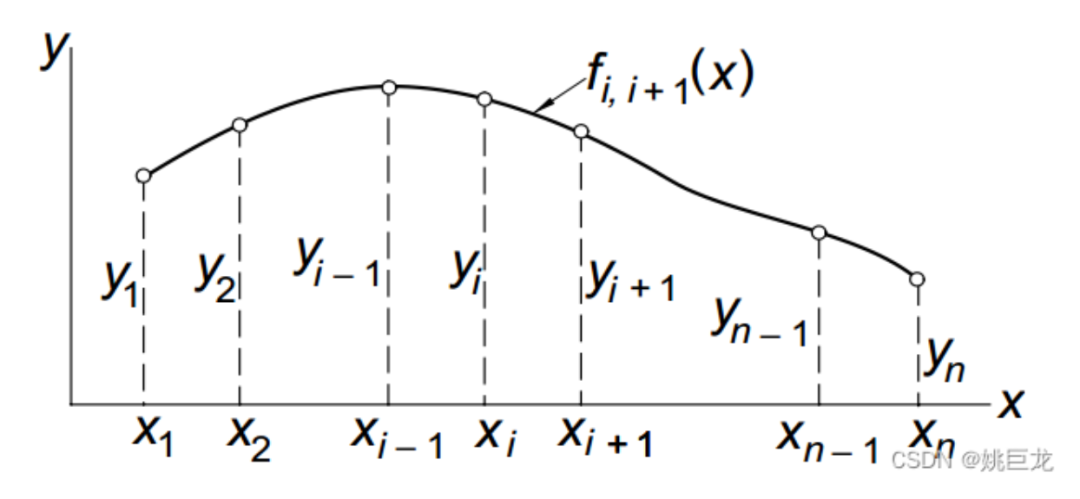RCS节点的数量比位置更重要。由于节点个数的选择和自由度有关, 所以当样本量比较大的时候可以取较多的节点。但是节点越多, 自由度越大, 模型越复杂, 越难解在「«Regression Modeling Strategies»」这本书中，Harrell 建议节点数为4时，模型的拟合效果较好，即同时可以兼顾曲线的平滑程度以及避免过拟合造成的精确度降低。当样本量较大时，5个节点是更好的选择。小样本（n<30）可以选择3个节点。当节点的个数为2时，得到的拟合曲线就是一条直线。大多数研究者推荐的节点为3-5个。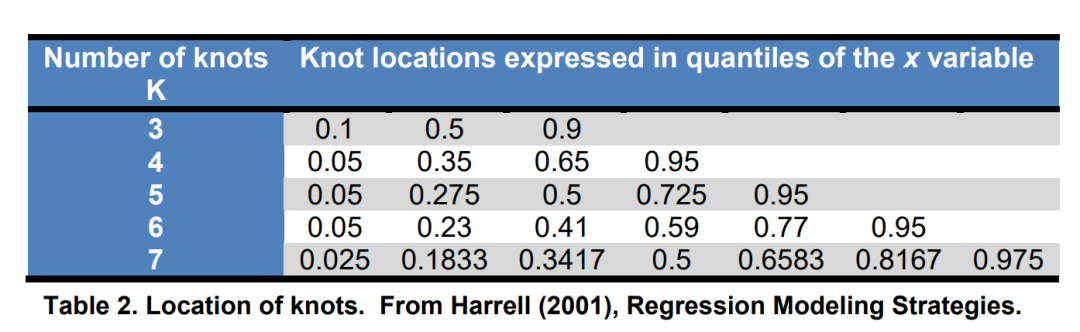## 一个例子

Lee DH, Keum N, Hu FB, et al. Predicted lean body mass, fat mass, and all cause and cause specific mortality in men: prospective US cohort study. BMJ. 2018;362:k2575. Published 2018 Jul 3. doi:10.1136/bmj.k2575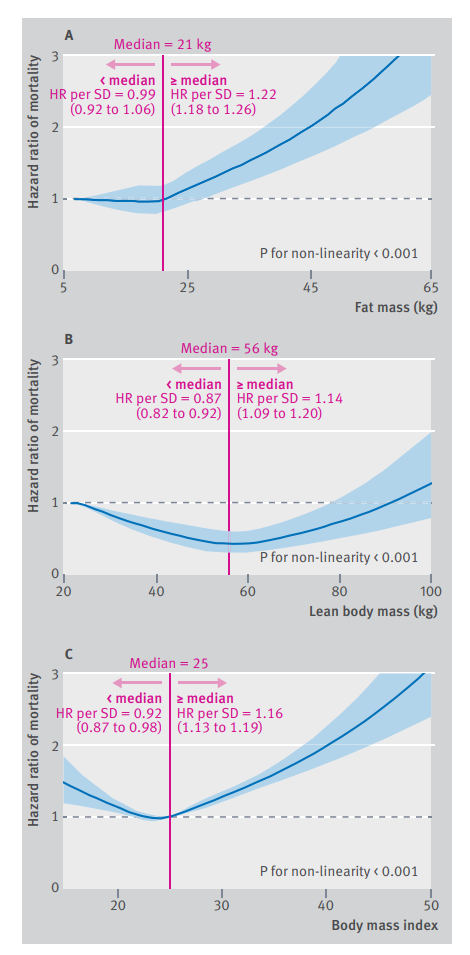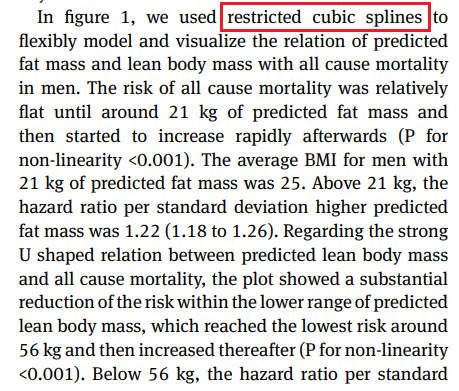如图，为了探究预测的FM、LBM和BMI与男性全因死亡率的关系，作者分别对这三个因素做了RCS分析。

## RCS实战

#加载所需要的包
library(ggplot2)
#install.packages('rms')
library(rms)
# 导入示例数据
head(data)
> head(data)
age    sex      time death
1 60.57519   Male  3.094579     1
2 42.11447   Male  1.574237     0
3 54.86611   Male  3.239313     0
4 55.82207   Male 12.495977     0
5 52.48256 Female  3.252534     0
6 46.12436   Male  2.836695     0
# 对数据进行打包，整理
dd <- datadist(data) #为后续程序设定数据环境
# 拟合模型
fit<- cph(Surv(time,death) ~ rcs(age,4) + sex,data=data)  # 节点数设为4
# 非线性检验
# P<0.05为存在非线性关系
anova(fit)
> anova(fit)
Wald Statistics          Response: Surv(time, death)

Factor     Chi-Square d.f. P
age        57.75      3    <.0001
Nonlinear  8.17      2    0.0168
sex        18.75      1    <.0001
TOTAL      75.63      4    <.0001
# 查看HR预测表
# 看一下预测的HR所对因的age
HR<-Predict(fit, age,fun=exp,ref.zero = TRUE)
head(HR)
> head(HR)
age  sex      yhat     lower    upper
1 19.71985 Male 0.7087866 0.2403429 2.090257
2 20.00869 Male 0.7052492 0.2429359 2.047356
3 20.29754 Male 0.7017294 0.2455536 2.005363
4 20.58638 Male 0.6982271 0.2481960 1.964258
5 20.87523 Male 0.6947423 0.2508632 1.924024
6 21.16408 Male 0.6912750 0.2535552 1.884643

Response variable (y):

Limits are 0.95 confidence limits
# 绘图
ggplot()+
geom_line(data=HR, aes(age,yhat),
linetype="solid",size=1,alpha = 0.7,colour="#0070b9")+
geom_ribbon(data=HR,
aes(age,ymin = lower, ymax = upper),
alpha = 0.1,fill="#0070b9")+
theme_classic()+
geom_hline(yintercept=1, linetype=2,size=1)+
geom_vline(xintercept=48.89330,size=1,color = '#d40e8c')+ #查表HR=1对应的age
labs(title = "Stroke Risk", x="Age", y="HR (95%CI)")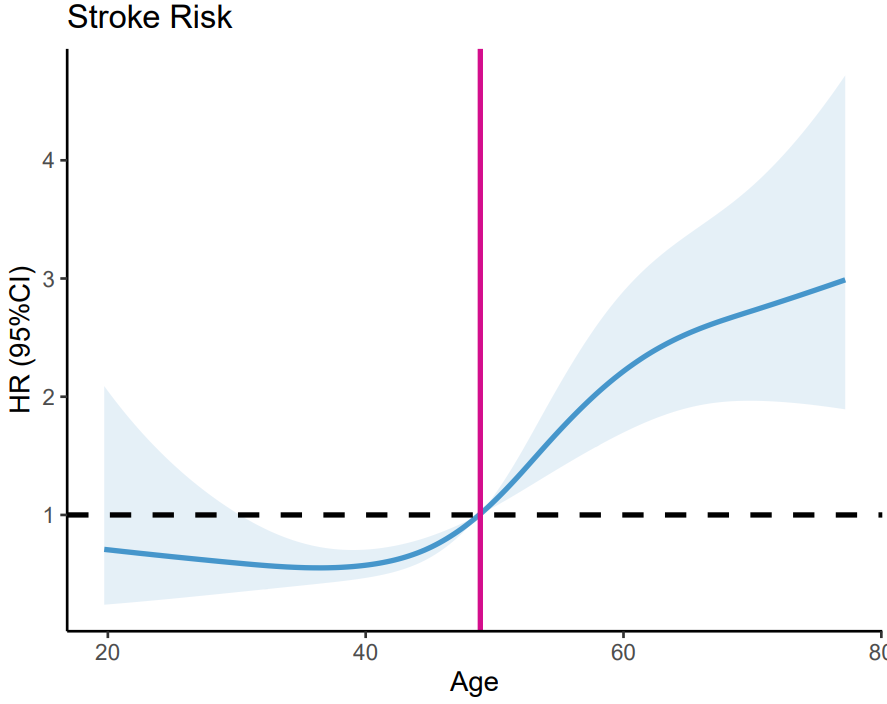可以看到，年龄<49岁，死亡风险随年龄变化不是很明显；年龄>49岁后，死亡风险随年龄的增加而增加。

### 分组

### 性别分组
HR1 <- Predict(fit, age, sex=c('Male','Female'),
fun=exp,type="predictions",
ref.zero=TRUE,conf.int = 0.95,digits=2)
HR1
ggplot()+
geom_line(data=HR1, aes(age,yhat, color = sex),
linetype="solid",size=1,alpha = 0.7)+
geom_ribbon(data=HR1,
aes(age,ymin = lower, ymax = upper,fill = sex),
alpha = 0.1)+
scale_color_manual(values = c('#0070b9','#d40e8c'))+
scale_fill_manual(values = c('#0070b9','#d40e8c'))+
theme_classic()+
geom_hline(yintercept=1, linetype=2,size=1)+
labs(title = "Stroke Risk", x="Age", y="HR (95%CI)")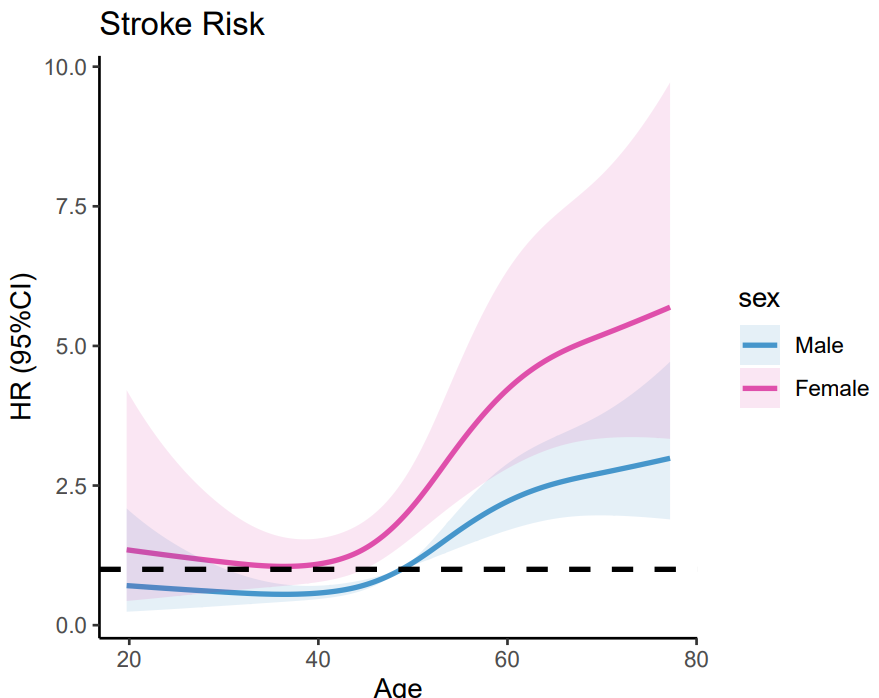## 示例数据和代码领取

点赞在看 本文，分享至朋友圈集赞25个保留30分钟，截图发至微信mzbj0002领取。

「木舟笔记2022年度VIP可免费领取」

### 木舟笔记2022年度VIP企划

「权益：」

1. 「2022」年度木舟笔记所有推文示例数据及代码(「在VIP群里实时更新」)。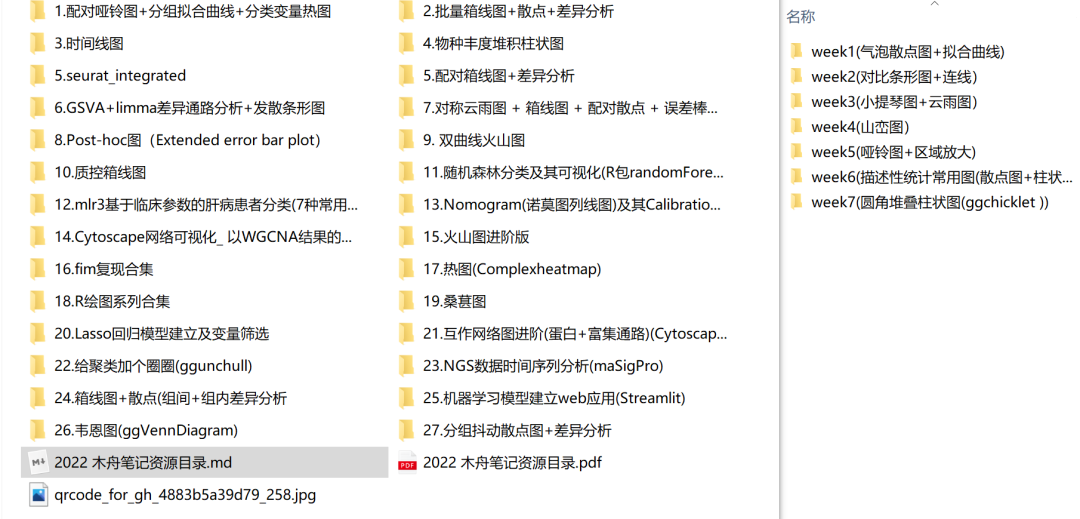2. 木舟笔记「科研交流群」

3. 「半价」购买跟着Cell学作图系列合集(免费教程+代码领取)|跟着Cell学作图系列合集

「收费：」

「99￥/人」。可添加微信：mzbj0002 转账，或直接在文末打赏。## 参考

1. https://blog.csdn.net/weixin_43645790/article/details/125285467

2. 限制性立方样条图，一种美的不行的趋势性分析方法（附R语言详细教程

3. Restricted cubic splines. A spline is a drafting tool for drawing… | by Peter Flom | Towards Data Science

4. R语言绘制限制性立方样条（Restricted cubic spline，RCS） - 简书 (jianshu.com)

## 往期内容展开全文• 相关介绍： 在病因推断、剂量效应研究中，时常要分析自变量和因变量的数量关系。广义线性模型，如Logistic回归、Possion回归等是应用比较广泛的方法。它的一个重要假设是通过...限制性立方样条中节点的个数(k)和...
• R语言使用cph函数和rcs函数构建限制性立方样条cox回归模型人工智能 机器学习 数据挖掘 r语言
• 因此，一个更好的解决方法是拟合自变量与因变量之间的非线性关系，限制性立方样条(Restricted cubic spline，RCS)就是分析非线性关系的最常见的方法之一。 最近有粉丝问有序多分类变量可以应用在限制立方条图吗？是...
• R语言如何确认限制性样条分析的最佳节点个数、进行方差分析通过p值确认指定连续变量和风险值HR之间是否存在非线性关系、限制性立方样条cox回归模型数据挖掘 人工智能 机器学习 r语言
• 一、背景介绍 最近在研究怎么处理论文数据，各种分析软件都有使用，比如：SPSS、Origin、stata16、medcalc和R语言都有些研究，其中除R语言外都是收费的。不过经过一番功夫，我这边有SPSS、stata16、Origin和medcalc...r语言 python
• 因此，一个更好的解决方法是拟合自变量与因变量之间的非线性关系，限制性立方(Restricted cubic spline，RCS)就是分析非线性关系的最常见的方法之一。 既往文章《stata两种方法制作限制立方条图》中，我们已经介绍了...
• R语言使用cph函数和rcs函数构建限制性立方样条cox回归模型、使用ggcoxzph函数可视化进行Schoenfeld残差图检验模型是否满足等比例风险数据挖掘 人工智能 机器学习 r语言
• R语言使用Predict函数计算指定连续变量和风险比HR值的关系、基于限制性立方样条分析方法、限制性立方样条cox回归模型数据挖掘 人工智能 机器学习 r语言
• R语言限制性立方样条(RCS, Restricted cubic spline)分析：基于logistic回归模型、南非心脏病数据集（South African Heart Disease） 目录 R语言限制性立方样条(RCS, Restricted cubic spline)分析：基于...r语言 数据挖掘 机器学习 人工智能
• 请问R中怎么看限制立方样条曲线与HR=1的截点?请问应该输什么代码看交点数据呢？r语言 线性回归 数据结构
• R语言使用cph函数和rcs函数构建限制性立方样条cox回归模型、使用rms包的Predict函数计算指定连续变量在不同分组变量下和风险比HR值的关系、使用ggplot2可视化连续变量在不同分组变量下和风险值HR的关系、美化可视化...数据挖掘 人工智能 机器学习 r语言
• R语言使用cph函数和rcs函数构建限制性立方样条cox回归模型、使用cox.zph函数执行PH检验、检验模型是否满足等比例风险
• 想问一下立方样条曲线的置信区间的问题，我应该怎么调整置信区间的图像成喇叭形，代码奉上，第一张是自己画的图，第二张是文献的图。 library(ggplot2) library(survival) library(rms) setwd("D:/work/work/zhang 9...r语言
• 限制性立方样条函数（RCS）在比较非线性关系中很常用。既往我们已经讲过R语言制作限制性立方条图，但是讲得比较简单，中间有些环节没写出来，我也不是很满意，今天重新来说一下。主要是要用到rms包的rcs函数来绘制，...大数据
• 因此，一个更好的解决方法是拟合自变量与因变量之间的非线性关系，限制性立方(Restricted cubic spline，RCS)就是分析非线性关系的最常见的方法之一。 既往教程中我们介绍了使用R语言在COX回归模型基础上绘制限制...大数据
• Fit就是你的模型，目前只支持cox回归和logistic回归，因为依托于RMS包的rcs函数支持，所以模型的格式是lrm和cph，等下我会演示怎么操作。Histbinwidth直方图宽度，默认是0.8，histcol直方图颜色，默认是绿色，...
• R语言使用cph函数和rcs函数构建限制性立方样条cox回归模型、使用rms包的Predict函数计算指定连续变量和风险比HR值的关系、使用ggplot2可视化连续变量和风险值HR的关系、美化可视化图像数据挖掘 人工智能 机器学习 r语言
• # 每一季的LOESS平滑 LOESS(se = FALSE) + goln(y = tmu,neyp= dhe"+ as(colo = sason) # 对去趋势的数值进行LOESS平滑处理 smooth(se = FALSE) + eoin(es =memu)), itype = ") + # 立方样条 g + smooth( "m", ns(x...r语言 LOESS
• R语言使用cph函数和rcs函数构建限制性立方样条cox回归模型、检验模型是否满足等比例风险、是否存在非线性关系、使用rms包的Predict函数计算指定连续变量和风险比HR值的关系并可视化数据挖掘 人工智能 机器学习 r语言
• ## stata两种方法制作限制立方条图

千次阅读 热门讨论 2021-04-02 15:24:22
我们前面已经讲了怎么使用R语言制作限制立方条图，今天来讲讲怎么使用stata制作限制立方条图，参考了网上常见的两种做法，顺便比较一下做图出来的差异，然后在和R语言比较一下。 首先要导入stata的xblc包，我们可以...大数据
• R语言使用ggplot2包可视化指定连续变量和风险比HR值的关系、、基于限制性立方样条分析方法、限制性立方样条cox回归模型数据挖掘 人工智能 机器学习 r语言
• 细节都差不多，想详细了解请看文章《首发： ggrcs包，一个用于绘制直方图+限制立方样条+双坐标轴图的R包》，如果你对结果不放心，想了解绘图原理，请看文章《R语言ggplot绘制直方图+限制立方样条+双坐标轴图》。...r语言 人工智能
• 作者： GURCHETAN SINGH 翻译：张逸 校对：丁楠雅本文共5800字，建议阅读8分钟。本文从线性回归、多项式回归出发，带你用Python实现样条回归。我刚开...